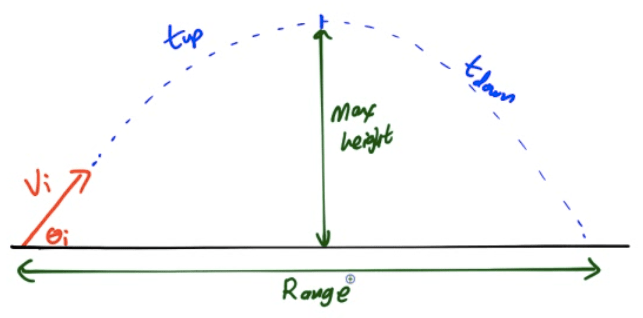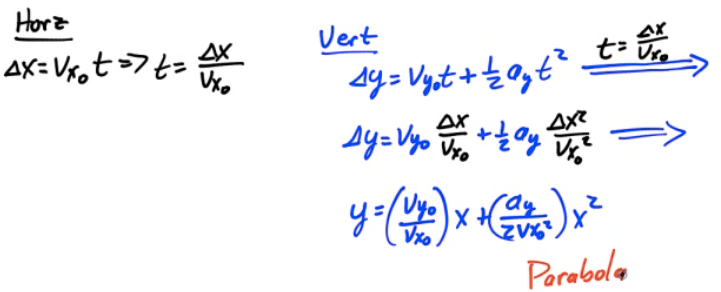# What Is a Projectile

• A projectile is an object that is in acted upon only by gravity

• In reality, air resistance plays a role

• Typically, projectiles are objects launched at an angle

# Path of a Projectile

• Projectiles launched at an angle move in parabolic arcs# Independence of Motion

• Projectiles launched at an angle have motion in two dimensions

• Vertical - like free fall

• Horizontal - 0 acceleration

• Vertical motion and horizontal motion can be treated separately!

# Angled Projectiles

• For objects launched at an angle, you must first break up the object's initial velocity into x and y components of initial velocity

• Then, use these components of initial velocity in your horizontal and vertical motion tables

• An object will travel the maximum horizontal distance with a launch angle of 45°# Graphing Projectile Motion

• Track vector components as a function of time

• Solve for time in the horizontal, and use that to eliminate time in the vertical equation